Courses

# Test: Electromagnetic Radiations

## 16 Questions MCQ Test Chemistry for JEE | Test: Electromagnetic Radiations

Description
This mock test of Test: Electromagnetic Radiations for JEE helps you for every JEE entrance exam. This contains 16 Multiple Choice Questions for JEE Test: Electromagnetic Radiations (mcq) to study with solutions a complete question bank. The solved questions answers in this Test: Electromagnetic Radiations quiz give you a good mix of easy questions and tough questions. JEE students definitely take this Test: Electromagnetic Radiations exercise for a better result in the exam. You can find other Test: Electromagnetic Radiations extra questions, long questions & short questions for JEE on EduRev as well by searching above.
QUESTION: 1

### Direction (Q. Nos. 1-8) This section contains 8 multiple choice questions. Each question has four choices (a), (b), (c) and (d), out of which ONLY ONE option is correct.   Q. FM radio broadcasts at 900 kHz. What wavelength does this corresponds to?

Solution: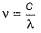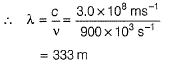QUESTION: 2

### The nucleus of helium contains

Solution:

A helium nucleus is made of two protons and two neutrons and has a charge +2.

QUESTION: 3

### Moseley’s equation is represented as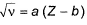where, a and b are constants.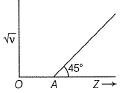Q. If OA = 1, then atomic number of the element showing frequency of 400 Hz is

Solution: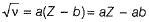Thus, graph is a straight line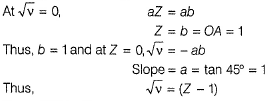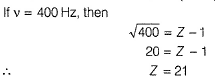QUESTION: 4

A gas absorbs photon of wavelength 355 nm and emits at two wavelengths. If one of the emissions is at 680 nm, the other is at

[AlEEE 2011]

Solution:

Absorbed energy = Emitted energy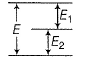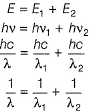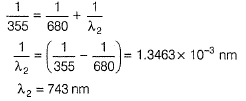QUESTION: 5

The longest wavelength of light capable of breaking a single (Cl — Cl) bond in Cl2 is if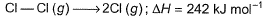[AlEEE 2010]

Solution:

ΔH = 242 kJ mol-1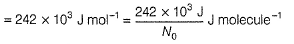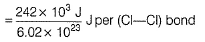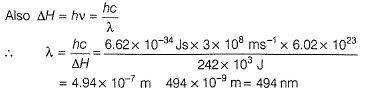QUESTION: 6

Which of the following conclusions could not be derived from Rutherford’s α-particle scattering experiment?

Solution:

Option A: Electrons (-) and nucleus (+) are held together by electrostatic forces of attraction.
Option B: Radius of the atom (= 10-10m) > radius of the nucleus (10-15m).
Option C: Most of the space is empty as given by the radius of the nucleus and atom.
Option D: As electron moves in a circular path, it emits energy and ultimately falls into the nucleus.

Thus, the correct option is D.

QUESTION: 7

Which of the following statements is not correct about the characteristics of cathode rays?

Solution:

(d) Cathode rays consist of negatively charged material particles called electron. They were discovered by William Crookes. The characteristics of cathode rays do not depend upon the material of electrodes and the nature of the gas present in the cathode ray tube.

QUESTION: 8

Energy of a mole of radio wave photons with a frequency of 909 kHz is

Solution:

E = N0hv
⇒ E = 6.02 x 1023 x 6.62 x 10-34 x 909 x10= 3.62 x 10-4 J

*Multiple options can be correct
QUESTION: 9

Direction (Q. Nos. 9-11) This section contains 3 multiple-choice questions. Each question has four choices (a), (b), (c), and (d), out of which ONE or  MORE THANT ONE  is correct.

Q. Select the correct statement(s).

Solution:

Option A: Oscillations travel through space at a velocity of light, thus electromagnetic radiation is in the form of energy E = hv.
Option B: Radiowaves, microwaves, X-rays, and visible light are part of the Electromagnetic radiation spectrum.
Option C: Some of the radiations are absorbed in the atmosphere, hence the spectrum differs.
Option D: Ozone layer absorbs ultraviolet radiation.

Hence, options A, B, C, and D are correct.

*Multiple options can be correct
QUESTION: 10

Consider the following transitions n = 1 to n = 2 and then, n = 2 to n = 3.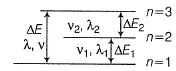Then

Solution:

Energy values are additives i.e.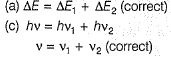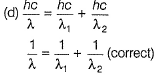Hence, options A, C, D are correct.

*Multiple options can be correct
QUESTION: 11

Select the correct statement(s).

Solution:

Option A: The ideal body, which emits and absorbs all frequencies is called a black body
Option B:  As temperature increases, distribution of frequency (and energy) increases.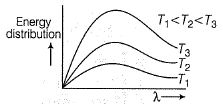Option C: As temperature increases, radiation emitted is shifted to a lower wavelength, i.e. higher frequency.
Option D: All types of radiations have the same speed 3 x 108 ms-1.

Hence, options A, B, C, and D are correct.

QUESTION: 12

Direction (Q. Nos. 12 and 13) This section contains a paragraph, wach describing  theory, experiments, data etc. three Questions related to paragraph have been  given.Each question have only one correct answer among the four given  ptions  (a),(b),(c),(d)

A hypothetical electromagnetic wave is pictured here.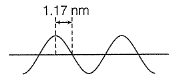Q. Energy associated with this wave is

Solution: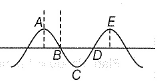A to E makes one complete wave.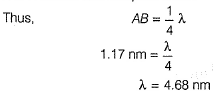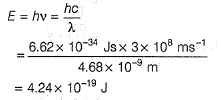QUESTION: 13

A hypothetical electromagnetic wave is pictured here.Q. A standing wave in a string 42 cm long has a total of six nodes (including those at the ends). Wavelength of the standing wave is

Solution:A to E makes one complete wave.If there are n nodes including those at the ends, in a string of length /, then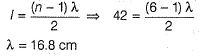QUESTION: 14

Direction (Q. Nos. 14) Choice the correct combination of elements and column I and coloumn II are given as option (a), (b), (c) and (d), out of which ONE option is correct.

Q. The colours of visible light are at different wavelength. Match the wavelengths in Column I with their colours in Column II.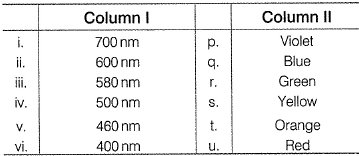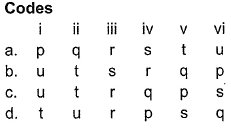Solution: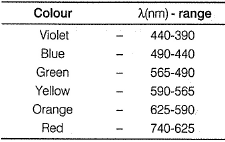Thus, (i)(700nm) Red (u)
(ii) (600 nm) - Orange (t)
(iii) (580 nm) - Yellow (s)
(iv) (500nm) - Green (r)
(v) (460 nm) - Blue (q)
(vi) (400 nm) - Violet (p)

*Answer can only contain numeric values
QUESTION: 15

Direction (Q. Nos. 15 and 16) This section contains 2 questions. when worked out will result in an integer from 0 to 9 (both inclusive)

Q. In Millikan’s experiment, the static electric charge on the oil drops has been obtained by shining X-rays. If the static electric charge on the oil drop is -1.282x 10-18 C, calculate the number of electrons present on it.

Solution:

Charge on one electron = - 1.6022 x 10-19 C
Let, number of electrons = N
Then total charge = - N x 1.6022 x 10-19C
Given, total charge = -1.282 x 10-18 C
Thus, - N x 1.6022 x 10-19 C = - 1. 282 x 10-18C

∴ N = 8

*Answer can only contain numeric values
QUESTION: 16

Energy change associated per mole of atoms with an atomic transition giving rise to radiations of 1 Hz is x * 10-10 J/mol. What is the value of x?

Solution:

Energy = n.hv
where,
n = 1 mole or 6.023 × 1023 atoms
h = 6.626×10-34 J.sec
v (frequancy) = 1 Hz

⇒ E = 6.023 ×1023 × 6.626×10-34 × 1 = 3.91 × 10-10
so approximately value of x = 4.